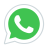# Basic Fundamentals Of Electricity And DC Circuit Analysis

2023-01-06 11:16:01The aim of this course is to provide a complete overview of the analysis of electrical circuits used in Electrical engineering and electronics. Circuit analysis is the most fundamental concept in electrical, electronic, and computer engineering. For this reason, circuit analysis is usually the first course taught in university programs in electrical, electronic, and computer engineering, since basically everything related to electrical, electronic, or computer engineering is based on circuit analysis.

## Electrical circuits and electronics

In this course, you will learn everything about electrical circuits and electronics, from the basics, such as electrical circuits, through electrical quantities, such as voltage, current, and power, to complex methods of analyzing electrical and electronic circuits. . The course is broadly divided into the following sections:

### Introduction to the course

Fundamentals of electrical circuits:

We will discuss what an electrical circuit is at the most elementary level, and then explain electrical quantities and sources of electricity. This is the basis of electrical engineering and electronics.

•Analysis of elementary DC circuits:

We will discuss the analysis of DC (direct current) circuits, starting with basic analytical techniques such as Kirchhoff's voltage law and Kirchhoff's current law (KVL and KCL), voltage distribution, current distribution, nodal analysis, and loop analysis. We will also discuss how complex resistor circuits can be simplified into equivalent circuits to simplify the analysis of circuit diagrams and electronics.

We will discuss advanced methods for the analysis of electrical circuits and electronics, such as the superposition theorem, Venin's theorem, and Norton's theorem.

•Devices for storing energy in electric circuits:

We will discuss passive components of electric circuits that are capable of storing energy: capacitors and inductors. We will discuss the basics of capacitors and inductors, how they store energy, and how to simplify complex circuits containing combinations of capacitors and inductors into simpler circuits to facilitate the analysis of circuits and electronics.

•Transients in electrical circuits:

We will discuss the analysis of first-order electrical circuits during transients. Things get a bit more complicated here, but we will solve some examples that illustrate how circuits behave during transients, in contrast to the steady-state circuits discussed earlier.

### Characteristics of a circuit

A circuit, which can be AC or DC, is a combination of active elements (power supplies) and passive elements (resistors, capacitors, and inductors). Thus, circuit theory or analysis helps to understand the behavior or characteristics of a circuit by finding the voltages and currents in the different elements of the circuit using various techniques. Thus, we will briefly discuss the basic concepts of electricity before dealing with DC circuit theory in the following articles.

### Niels Bohr's atomic model

According to the atomic theory, every material is made up of atoms. This atom consists of a centrally charged nucleus surrounded by electrons, based on Niels Bohr's atomic model. The atomic nucleus is made up of neutrons and positively charged protons. Lyskills provides  Basic Fundamentals Of Electricity And DC Circuit Analysis training courses in Lahore.  The electrons are negatively charged particles that rotate around the nucleus. In this atom, there are an equal number of protons and electrons, and there is a strong attractive force between their opposing charges that forces the electrons to follow the nucleus.